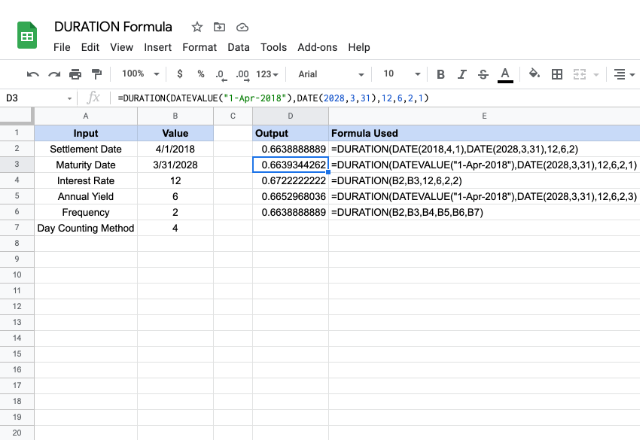# How to use the DURATION formula in Google SheetsDo you need to calculate the number of compounding periods that an investment required to reach a target value? The DURATION formula in Google Sheets can help us with that. It considers the present value of an investment as well as the rate of appreciation to arrive at the duration.

### Syntax

DURATION(settlement, maturity, rate, yield, frequency, [day_count_convention])

• settlement – is the date after issuing the security, when it is actually delivered to the buyer.
• maturity – is the end or maturity date of the security, when the buyer can redeem it at face or par value.
• rate – is the annualized interest rate at which the investment appreciates.
• yield- is the annual yield that the buyer expects for the security.
• frequency – is the number of interest payments that buyer can pay per year.
• day_count_convention – [OPTIONAL parameter – 0 by default ] – it is an indicator of the day count method that Google Sheets should consider. There are five different possible values for this parameter.
• 0 – assumes that there are 30 day months and 360 day years (US (NASD) 30/360). Using this value ensures there are specific adjustments to the entered dates that usually are at the end of months.
• 1 – calculates based on the actual number of days between the specified dates, and the actual number of days in the intervening years.
• 2 – calculates based on the actual number of days between the specified dates, but assumes a 360 day year.
• 3 – evaluates the DURATION formula in Google Sheets based on the actual number of days between the specified dates, but assumes a 365 day year.
• 4 – very similar to the first option 0, except, it adjusts end-of-month dates according to European financial conventions.

### Usage: DURATION formula in Google Sheets

Examples make the understanding process much easier when learning new concepts. So, let go ahead and dive into a few of them. Please see the snapshot below.For settlement and maturity parameters, we need to ensure they get valid dates. They can either be results returned from formulas such as DATE, TO_DATE, or references to other date type cells. Google Sheets may return parsing errors if we enter direct text values for dates.

The other parameters – rate, yield, frequency, and day_count_convention – can be direct numeric values or can be references to the cells holding the appropriate values. This is demonstrated in the examples above.

We will observe that all the initial five parameters are the same essentially, yet the output values vary across the examples. This is due to the method with which the days are counted which we indicated using the day_count_convention parameter.

### DURATION formula

And there you go! Use the DURATION formula in Google Sheets to calculate the number of compounding periods that an investment required to reach a target value.

If you’d like to learn more about the various formulas of Google Sheets, why not take a look at our blog post on the MDURATION formula in Google Sheets.

Alternatively, check out related blog posts below!